### Home > MC2 > Chapter 1 > Lesson 1.1.3 > Problem1-20

1-20.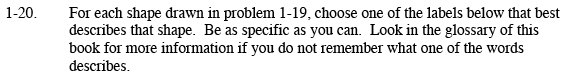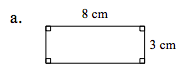This shape has two pairs of sides of equal lengths.

Notice that all four angles can be identified as right angles.

This shape must be a rectangle!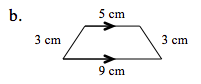Notice that there is one pair of parallel sides.

This must be a trapezoid.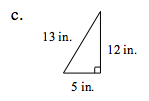This shape has 3 sides of unequal lengths.

Notice that there is one right angle.

Possible names for this shape are scalene triangle and right triangle.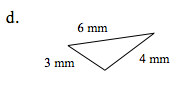All three sides of this triangle are of unequal lengths.

One of the three angles is greater than ninety degrees.

Possible names for this shape are scalene triangle and obtuse triangle.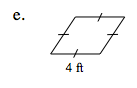Notice that all 4 sides of this shape are of equal lengths.

But there are no right angles, so this shape cannot be a square.

It must be a rhombus!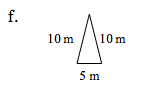Notice that this triangle has two sides of equal lengths.

This must be an isosceles triangle.